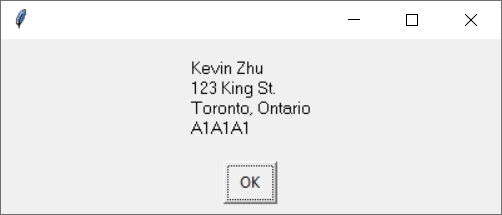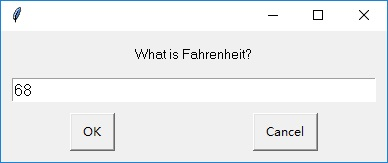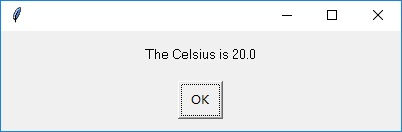TORONTO KIDS COMPUTER CLUB | Summer Camp 2021 Python Homework 16:30 – week 02
19829

# Summer Camp 2021 Python Homework 16:30 – week 02

## 14 Aug Summer Camp 2021 Python Homework 16:30 – week 02

Question 1.

Write a program that asks for your first name, then asks for your last name, and then prints a message with your first and last names in it.

```Sample Input:
What is your first name? Kevin
What is your Last name? Zhu

Sample Output:
(it means if user enters the above answer as Kevin for first name and Zhu for last name,
then the following information will be printed out)

```

Hint:

`firstName = input("What is your first name?")`

The string answer as value will be assigned to variable firstName.

Question 2.

Write a program that asks for the dimensions (length and width) of a rectangular room, and then calculates and displays the total amount(area) of carpet needed to cover the room.

```Sample Input:
Width: 5.2
Height: 2.4

Sample Output:
Carpet need: 12.48```

Hint:
Use input() to ask question and get answer, however, you can only get string data type of answer. In order to get decimal number as dimensions, you need to use float(input(…..)) which will convert from string answer into a decimal number, for example:

`h = float(input('Height: '))`

Question 3.

Write a program that helps the user add up her change. The program should ask:

• “How many quarters?”
• “How many dimes?”
•  “How many nickels?”

Then it should give the total value of the change

```Sample Input:
How many quarters? 1
How many dimes? 2
How many nickels? 4

Sample Output:
The total is \$0.65

```

Question 4:
Write a program to use easygui enterbox that asks for your name, then street, then city, then province, then postal code (all in easygui message boxes). The program should then display a mailing-style full address that looks something like this:```Hint:
Do not forget to import easygui in order to use enterbox and msgbox. You can type the following code to get the input from enterbox:
name = easygui.enterbox('What is your name?')

for example, if we type the following code:

print('Kevin\nZhu')

it will show Kevin and Zhu in two different lines
Kevin
Zhu

```

Question 5:

Use EasyGui to write a program to convert temperatures from Fahrenheit to Celsius. The formula for that is: Celsius = 5 / 9 * (Fahrenheit – 32). Use GUI input and output. You need to create a easygui enterbox to ask Fahrenheit, then use message box to show the Celsius degree. (Hint: the input you get from the enter box is a string, so you need float() to convert it into decimal number.Question 6:

A store is having a sale. They’re giving 10 percent off purchases of \$10 or lower, and 20 percent off purchases of greater than \$10. Write a program that asks the purchase price and displays the discount (10% or 20%) and the final price.

```Sample Input 1:
How much: 10

Sample Output 1:
You got 10% discount and your final price is \$9```

```Sample Input 2:
How much: 15.5

Sample Output 2:
You got 20% discount and your final price is \$12.4```

Question 7:

A soccer team is looking for girls from ages 10 to 12 to play on their team. Write a program to ask the user’s age and if male or female (using “m” or “f”). Display a message indicating whether the person is eligible to play on the team.

```Sample Input 1:
Age: 10
Gender: f

Sample Output 1:
Welcome to the team!```

```Sample Input 2:
Age: 10
Gender: m

Sample Output 2:
You are not allowed to play!```